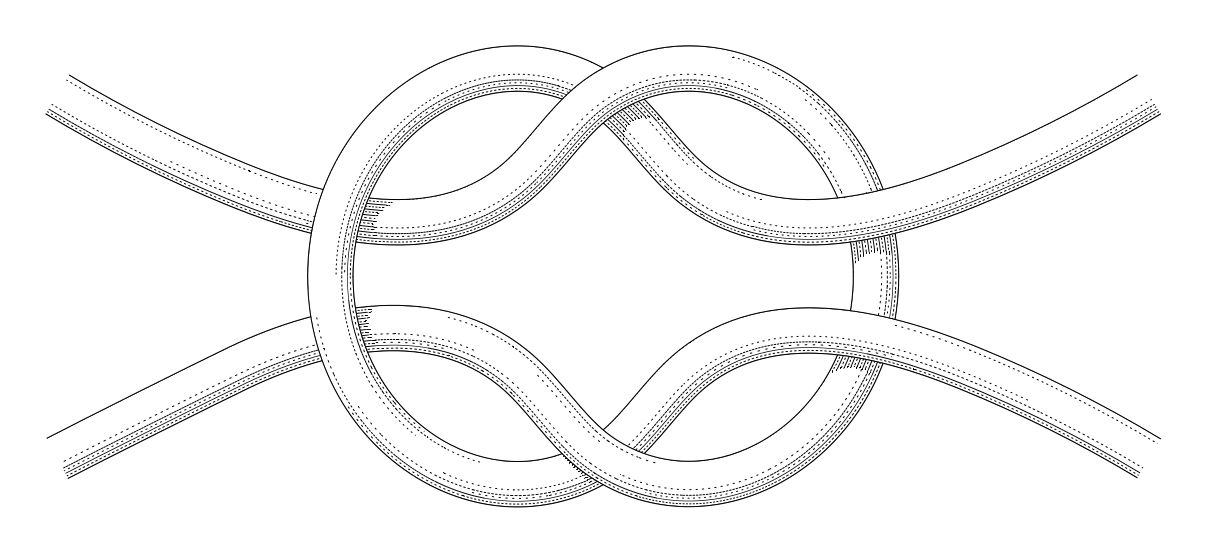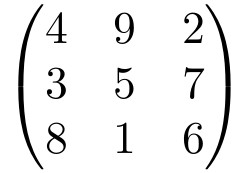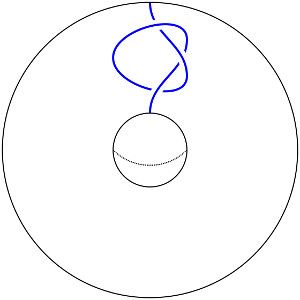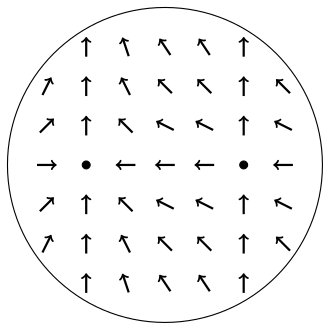# Teaching

## My Interests

My field of study is geometric topology. I am interested in geometric objects up to dimension 4 and their embeddings. In particular, I study knots and their mathematical properties. Here is an example of an interesting (part of a) knot:I am especially curious about concordance, a special equivalence relation on knots. This relation is intimately tied to questions on the topology of 4-manifolds and their smooth structures.

## ETH / Introduction to 3-manifolds

An introduction to the basic notions and tools of geometric topology with a special focus on three dimensional manifolds.

G26.5, Fridays 11:15

Course page

## McMaster / Instantons and 4-manifolds

We will go through the book of Freed and Uhlenbeck, and learn about Instantons and 4-manifolds and the involved analysis. This will culminate in a proof of Donaldson's diagonalisation theorem.

H312, Fridays 11:15

## McMaster / Math 1ZC3

Introduction to linear algebra for engineers. We cover linear independence, dimension, determinants, eigenvalues, diagonalisation, and complex numbers.## McMaster / Light bulbs in dimension 3 and 4

After recalling why light bulbs are standard in dimension 3, we go through Gabai's proof (ArXiv:1705.09989) of the corresponding statement in dimension 4. Along the way, we pick up the necessary background on embedded Morse theory and diffeomorphism groups.

H312, Fridays 13:00## McMaster / Math 731

Introduction to algebraic topology with focus on the fundamental group and homology.

Course page

## UQAM / SW stable homotopy theory

We study the approach to Seiberg-Witten theory of Bauer-Furuta using stable homotopy theory.

Seminar page## McGill / Math 123

Introduction to linear algebra with applications from probability theory and optimisation.

## McGill / Math 381

Complex calculus focusing on methods for evaluating integrals over domains in the complex plane and the computation of various transforms and their application in engineering.